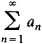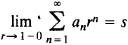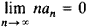Tauberian Theorem

Tauberian Theorem

If an infinite series is summable or an integral is integrable by a certain method, it is of interest to know under what conditions summability or integrability may be obtained for a weaker method (see). The conditions that must be imposed on the series or integral are established by theorems known as Tauberian theorems.

One of the first such theorems was proved by A. Tauber in 1897. It states that if for the series of numbersthere exists the limit(that is, if the series is summable to s by the Abel method) and ifthen the series converges to s.

Tauberian theorems are used in many fields of mathematics, particularly in analytic number theory and the study of the asymptotic behavior of the eigenvalues and eigenfunctions of differential operators.

REFERENCE

Hardy, G. Raskhodiashchiesia riady. Moscow, 1951. (Translated from English.)
References in periodicals archive ?
Yavuz, "Euler summability method of sequences of fuzzy numbers and a Tauberian theorem," Journal of Intelligent and Fuzzy Systems, p.
Maddox, "A Tauberian theorem for statistical convergence," Mathematical Proceedings of the Cambridge Philosophical Society, vol.
Since [T.sub.j] is almost surely nondecreasing in j, then x(j) is nondecreasing and hence a Tauberian theorem for power series yields the asymptotic equivalence
Canak and Totur  proved a Tauberian theorem for Cesaro summability methods, and Totur and Dik  gave some one-sided Tauberian conditions for a general summability method using the general control modulo of integer order.
by the well-known Tauberian theorem for Laplace transforms (see ).
Feichtinger, An elementary approach to Wiener's third Tauberian theorem for the Euclidean n-space.
After translating the above sums into a recurrence, we apply the Mellin transform and Tauberian theorem to discover that we need to handle infinite saddle points on a line (incidently, already encountered in (8)).
The text covers the Stieltjes integral, fundamental formulas, the moment problem, absolutely and completely monotonic functions, Tauberian theorems, the bilateral Laplace transform, inversion and representation problems for the Laplace transform, and the Stieltjes transform.
Indeed, Karamata-type Tauberian theorems would translate the asymptotics of B near zero into the the asymptotics of [M.sub.], n [right arrow] [infinity].

Site: Follow: Share:
Open / Close